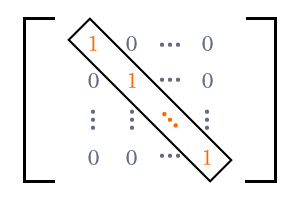Identity Matrix (Unit Matrix)Unit Matrix

A diagonal matrix whose diagonal elements are equal and $1$, is called an identity matrix.

Identity matrix is a square matrix and also a diagonal matrix. So, it is having elements only on principal diagonal but its non-diagonal elements are zero.

The meaning of identity is oneness. So, identity matrix is having elements on the leading diagonal but all the elements should be same. There is also another meaning for identity and it is unity. The meaning of unity is number $1$. Therefore, all diagonal elements are $1$ in an identity matrix. Hence, an identity matrix is also called as a unit matrix.

Identity matrix is denoted by a letter $I$ in mathematics. It is a square matrix. It means, the number of rows and number of columns are equal. Therefore, order of matrix is expressed as subscript to the letter $I$ as per the concept of order representation of square matrix. If number of rows and number of columns are $n$, the identity matrix is denoted by ${I}_{n}$ in mathematics.

${I}_{n}=\left[\begin{array}{ccccc}1& 0& 0& \cdots & 0\\ 0& 1& 0& \cdots & 0\\ 0& 0& 1& \cdots & 0\\ ⋮& ⋮& ⋮& \ddots & ⋮\\ 0& 0& 0& \cdots & 1\end{array}\right]$

An identity matrix ${I}_{n}$ is read in one of three following methods.

• An identity matrix of order $n$.
• ${n}^{th}$ order identity matrix.
• $n$ rowed identity matrix.

Example

${I}_{1}=\left[\begin{array}{c}1\end{array}\right]$

It is an identity matrix of order $1$.

${I}_{2}=\left[\begin{array}{cc}1& 0\\ 0& 1\end{array}\right]$

It is a second order identity matrix.

${I}_{3}=\left[\begin{array}{ccc}1& 0& 0\\ 0& 1& 0\\ 0& 0& 1\end{array}\right]$

It is a $3$ rowed identity matrix.

Latest Math Topics
Latest Math Problems
Email subscription
Math Doubts is a best place to learn mathematics and from basics to advanced scientific level for students, teachers and researchers. Know more